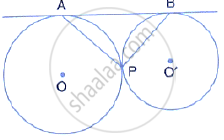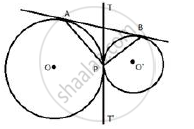Share

# In the Given Figure, Two Circles Touch Each Other Externally at Point P. Ab is the Direct Common Tangent of These Circles. Prove that Tangent at Point P Bisects Ab, - Mathematics

Course

#### Question

In the given figure, two circles touch each other externally at point P. AB is the direct common tangent of these circles. Prove thattangent at point P bisects AB,

#### SolutionDraw TPT' as common tangent to the circles.
i) TA and TP are the tangents to the circle with centre O.
Therefore, TA = TP ………(i)
Similarly, TP = TB ………..(ii)
From (i) and (ii)
TA = TB
Therefore, TPT' is the bisector of AB

Is there an error in this question or solution?